Welcome To Basics In Maths

# Differentiation(2m Q & S) || V.S.A.Q’S||## Differentiation(2m Q & S) || V.S.A.Q’S||

This content designed by the ‘Basics in Maths‘ team. These notes to do help the intermediate First-year Maths students.

Inter Maths – 1B two mark questions and solutions are very useful in IPE examinations.

### Differentiation

#### Question 1

Find f’ (x) for the following functions

(i) f(x) = (ax + b) (x > -b/a)

Sol:

Given f(x) = (ax + b) n

f’ (x) = n (ax + b) n – 1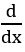(ax + b)

= n (ax + b) n – 1 a

= an (ax + b) n – 1

(ii)  f(x) = x2 2x log x

Sol:

Given f(x) = x2 2x log x

= 2×2x log x +x2 2x log a log x + x2 2x (1/x)

= x 2x[log x2 + x log x log 2 + 1]

Sol:

(iv) f(x) = log (sec x + tan x)

Sol:

Given, f(x) = log (sec x + tan x)

= sec x

#### Question 2

Find the derivative of  the following  functions

(i) f(x) = ex (x2 + 1)

Sol:

Given f(x) = ex (x2 + 1)

= ex (2x + 0) + (x2 + 1) ex

= ex (x2 + 2x + 1)

= ex (x + 1)2

(ii)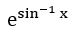(iii) cos (log x + ex)

(iv) x = tan (e-y)

e-y = tan-1 x

(v) cos [log (cot x)]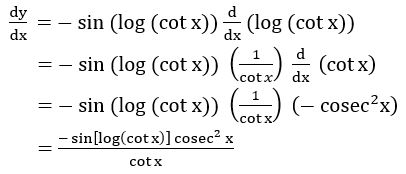(vi) sin[tan-1(ex)]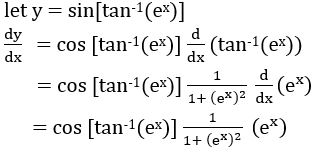(vii) cos-1(4x3 – 3x)

let y = cos-1(4x3 – 3x)

put x = cos θ ⟹ θ = cos-1 x

y = cos-1(4 cos 3 θ – 3cos θ)

= cos-1(cos 3θ)

= 3 θ

= 3 cos-1 x

(viii)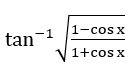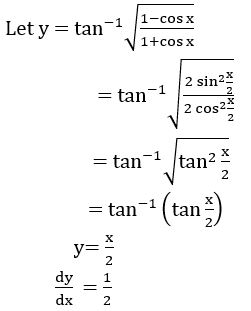(ix)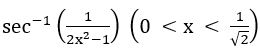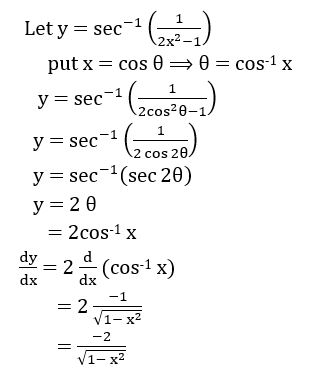(x)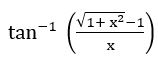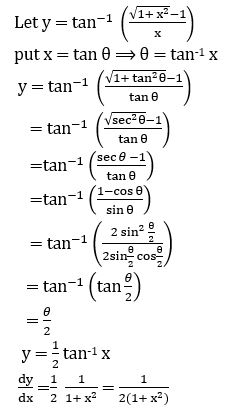#### Question 3

Find f’ (x), If f(x) = (x3 + 6 x2 + 12x – 13)100.

Sol:

Given f(x) = (x3 + 6 x2 + 12x – 13)100

f’ (x) = 100(x3 + 6 x2 + 12x – 13)99(x3 + 6 x2 + 12x – 13)

= 100(x3 + 6 x2 + 12x – 13)99 (3x2 + 12 x + 12 – 0)

=100(x3 + 6 x2 + 12x – 13)99 3 (x2 + 4 x + 4)

= 300 (x + 2)2 (x3 + 6 x2 + 12x – 13)99

##### Question 4

If f(x) = 1 + x + x2 + x3 + …. + x100, then find f’ (1).

Sol:

Given f(x) = 1 + x + x2 + x3 + …. + x100

f’(x) = 0 + 1 + 2x + 3 x2 + … 100 x99

f’(1) =  1 + 2 + 3 + … + 100

= 50 × 101

= 5050

#### Question 5

From the following functions. Find their derivatives.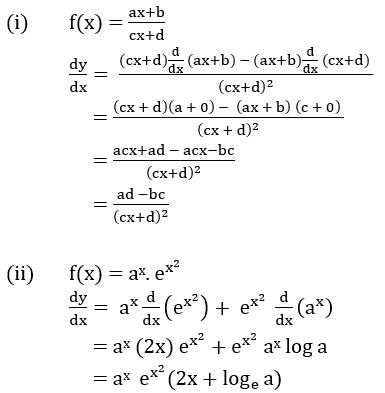#### Question 6

Sol: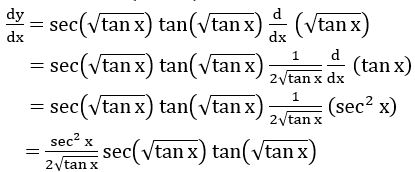##### Question 7

Sol:

Given y = log (cosh 2x)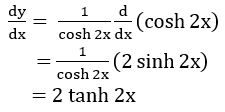##### Question 8

If x = a cos3 t, y = a sin3 t, find

Sol:

Given If x = a cos3 t, y = a sin3 t##### Question 9

Differentiate f(x) with respect to g(x) for the following.

derivative of f(x) with respect to g(x) =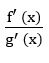put x = tan θ ⟹ θ = tan-1 x

##### Question 10

Sol: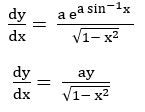#### Visit My Youtube Channel:  Click  on below  logo